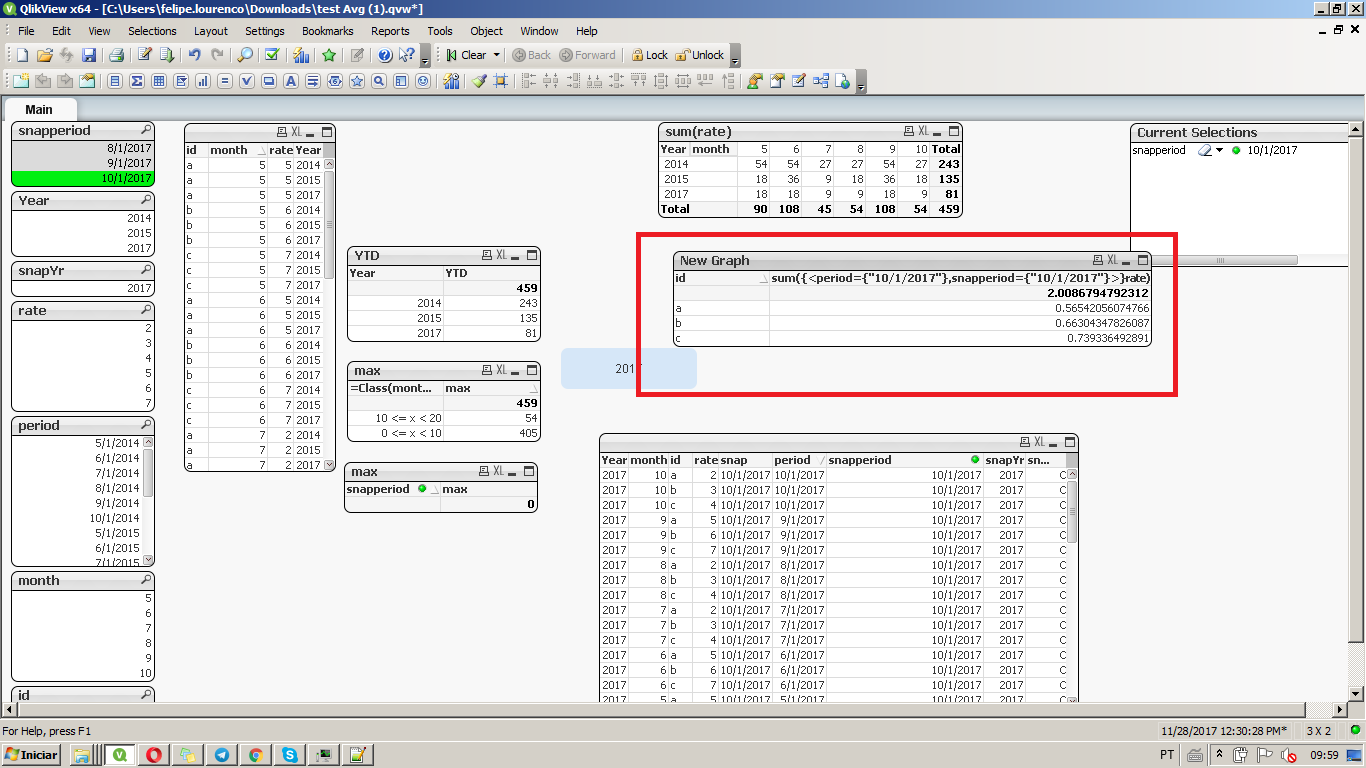# QlikView App Dev

Discussion Board for collaboration related to QlikView App Development.

cancel
Showing results for
Did you mean:Creator

## how? calculating % by dividing total for year by avg of totals from past months same fy year

how do i achieve this? I need to  calculate % by dividing total for year by avg of totals from past months same fy yearsample data file attached

1 Solution

Accepted SolutionsPartner

I guess you're missing the point of set analysis, if you combine both expression into one, it gets the same result, I just assumed you needed to see both values independently, but you can get only one expression with the two I put on the graph, giving the following:Set analysis let's you get a specific selection of data, even though there's a different selection made by the user.

Felipel.

3 RepliesPartner

Hi Rashid,

Both can be achieved through set analysis, being:

Column B (gets the max period and snapperiod or selected by user):

sum({<period={"\$(=max(period))"},snapperiod={"\$(=max(snapperiod))"}>}rate)

Column C (gets the year interval to max snapperiod or selected snapperiod:

avg({<snapperiod={">=\$(=Yearstart(max(snapperiod)))<=\$(=max(snapperiod))"}>}rate)

and Column 😧

// It only gets the previous stated expressions and does the percentage

Column(1)/Column(2)

Attachedt he file you provided with teh "New Graph" table, wich holds the above expression.

Felipe.Creator
Author

this works when columns are calculated separately, what if I have one expression in the pivot table and   different partial sum sort of like

Year | Color | STatus> Active | Inactive | Status Total | Year total

now in this I want to divide Status total /Year TotalPartner

I guess you're missing the point of set analysis, if you combine both expression into one, it gets the same result, I just assumed you needed to see both values independently, but you can get only one expression with the two I put on the graph, giving the following:Set analysis let's you get a specific selection of data, even though there's a different selection made by the user.

Felipel.